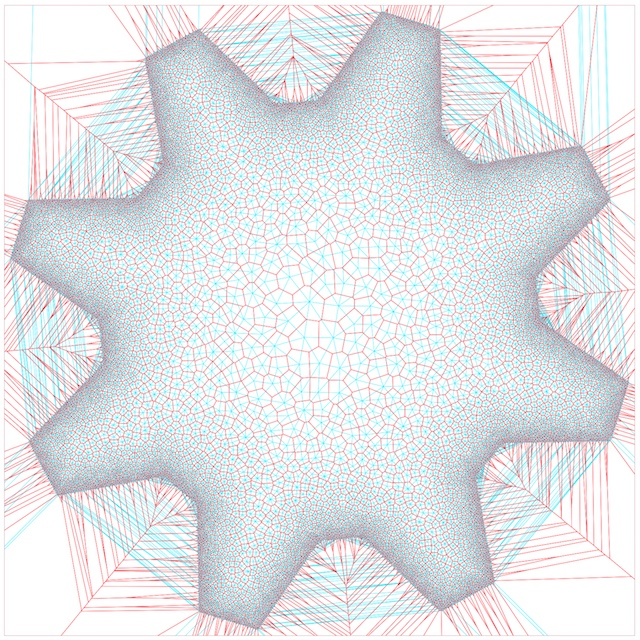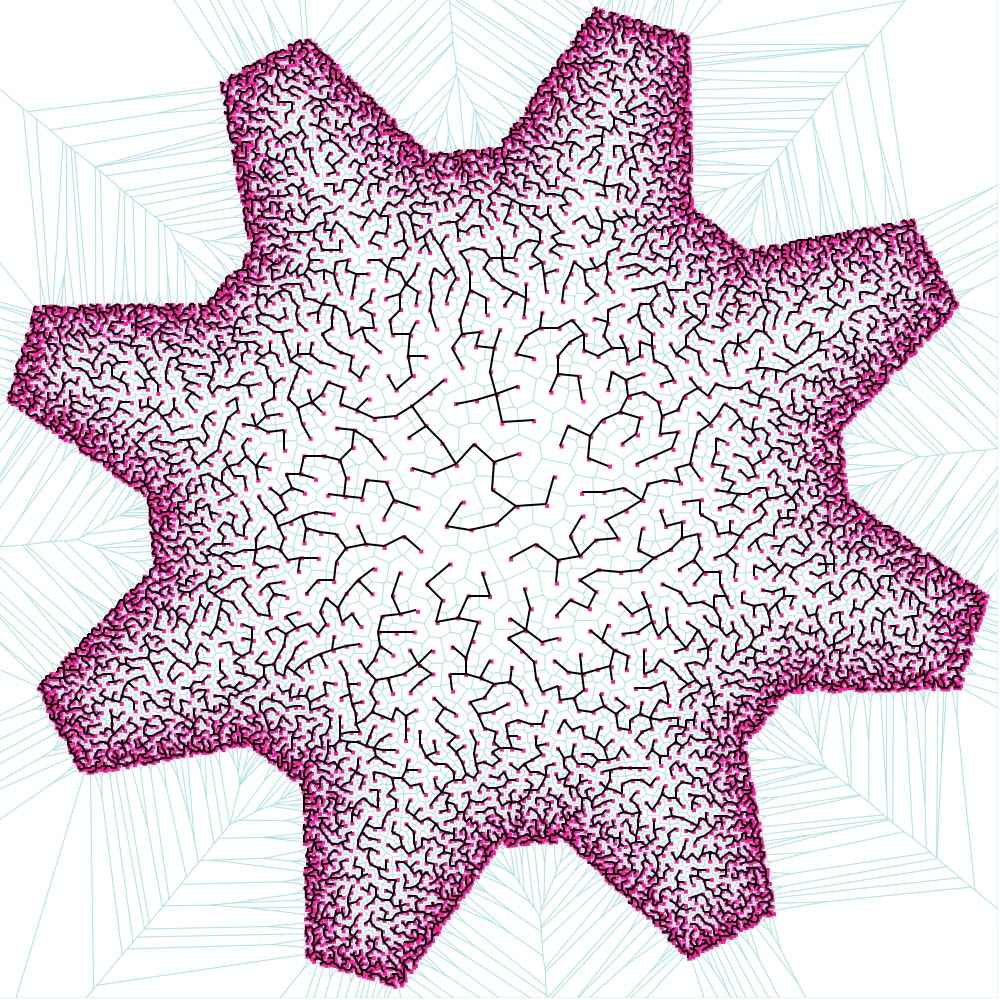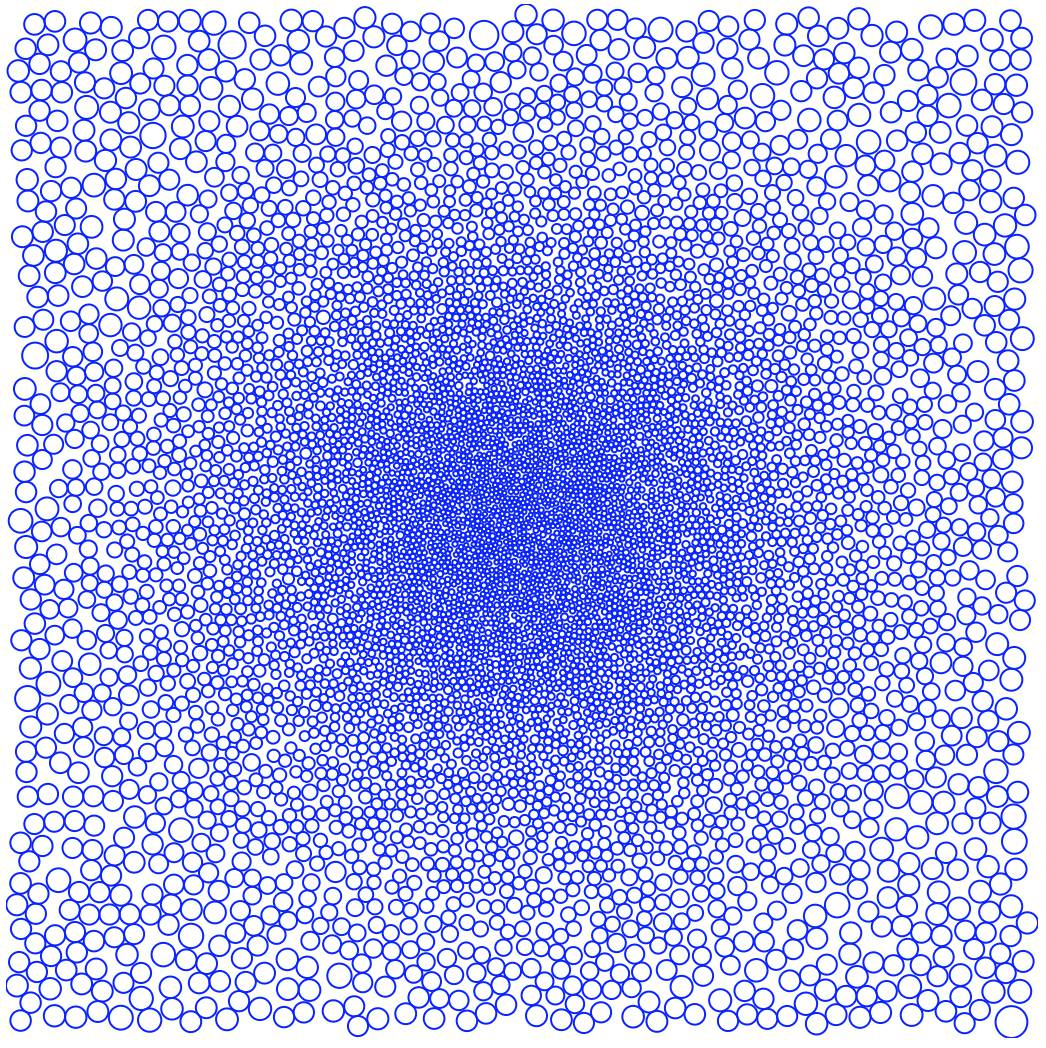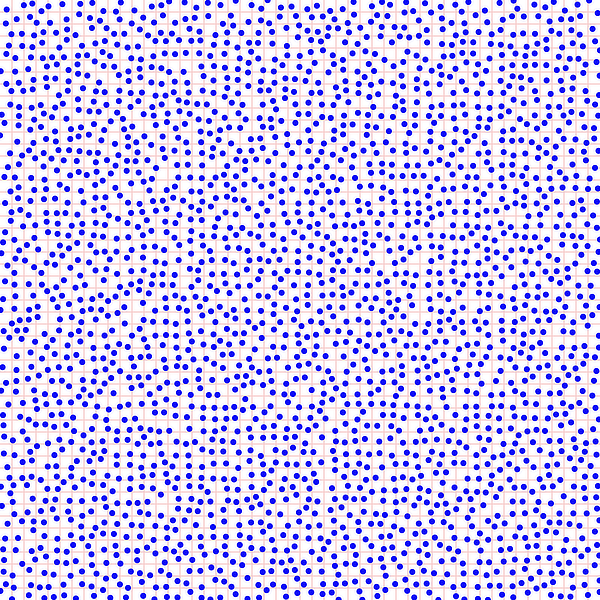Options
All
• Public
• Public/Protected
• All

#This project is part of the @thi.ng/umbrella monorepo.nD Stratified grid and Poisson disc sampling with support for variable spatial density, custom PRNGs (via @thi.ng/random's `IRandom` interface & implementations) and customizable quality settings.

The Poisson disc sampler requires a spatial index and we recommend using `KdTreeSet` from the @thi.ng/geom-accel package to speed up the sampling process, but other `ISpatialSet`-compatible indices are supported as well...

### Status

STABLE - used in production

Search or submit any issues for this package

## Installation

``yarn add @thi.ng/poisson``
``````// ES module
<script type="module" src="https://unpkg.com/@thi.ng/poisson?module" crossorigin></script>

// UMD
<script src="https://unpkg.com/@thi.ng/poisson/lib/index.umd.js" crossorigin></script>``````

Package sizes (gzipped, pre-treeshake): ESM: 455 bytes / CJS: 514 bytes / UMD: 631 bytes

## Usage examples

Several demos in this repo's /examples directory are using this package.

A selection:

ScreenshotDescriptionLive demoSourcePoisson-disk shape-aware sampling, Voronoi & Minimum Spanning Tree visualizationDemoSource2D Poisson-disc sampler with procedural gradient mapDemoSource2D Stratified grid sampling exampleDemoSource

## API

Generated API docs

### Poisson disc sampling

The package provides a single function `samplePoisson()` and the following options to customize the sampling process:

• points: Point generator function. Responsible for producing a new candidate point within user defined bounds using provided RNG.
• density: Density field function. Called for each new candidate point created by point generator and should return the poisson disc exclusion radius for the given point location. The related candidate point can only be placed if no other points are already existing within the given radius/distance. If this option is given as number, uses this value to create a uniform distance field.
• index: Spatial indexing implementation for nearest neighbor searches of candidate points. Currently only @thi.ng/geom-accel types are supported. The data structure is used to store all successful sample points. Furthermore, pre-seeding the data structure allows already indexed points to participate in the sampling process and so can be used to define exclusion zones. It also can be used as mechanism for progressive sampling, i.e. generating a large number of samples and distributing the process over multiple invocations of smaller sample sizes (see `max` option) to avoid long delays.
• max: Max number of samples to produce. Must be given, no default.
• jitter?: Step distance for the random walk each failed candidate point is undergoing. This distance should be adjusted depending on overall sampling area/bounds. Default: 1
• iter?: Number of random walk steps performed before giving up on a candidate point. Increasing this value improves overall quality. Default: 1
• quality?: Number of allowed failed consecutive candidate points before stopping entire sampling process (most likely due to not being able to place any further points). As with the `iter` param, increasing this value improves overall quality, especially in dense regions with small radii. Default: 500
• rnd?: Random number generator instance. Default: @thi.ng/random `SYSTEM` (aka Math.random)``````import { asSvg, circle, svgDoc } from "@thi.ng/geom";
import { KdTreeSet } from "@thi.ng/geom-accel";
import { fit01 } from "@thi.ng/math";
import { samplePoisson } from "@thi.ng/poisson";
import { dist, randMinMax2 } from "@thi.ng/vectors";

const index = new KdTreeSet(2);

const pts = samplePoisson({
index,
points: () => randMinMax2(null, [0, 0], [500, 500]),
density: (p) => fit01(Math.pow(dist(p, [250, 250]) / 250, 2), 2, 10),
iter: 5,
max: 8000,
quality: 500,
});

// use thi.ng/geom to visualize results
// each circle's radius is set to distance to its nearest neighbor
const circles = pts.map((p) =>
circle(p, dist(p, index.queryKeys(p, 40, 2)) / 2)
);

document.body.innerHTML = asSvg(
svgDoc({ fill: "none", stroke: "blue" }, ...circles)
);``````

### Stratified grid sampling

The `stratifiedGrid` function can produce nD grid samples based on the following config options:

• dim: nD vector defining grid size (in cells)
• samples?: Number of samples per grid cell (default: 1)
• rnd?: Random number generator instance. Default: @thi.ng/random `SYSTEM` (aka Math.random)``````import { asSvg, circle, svgDoc } from "@thi.ng/geom";
import { KdTreeSet } from "@thi.ng/geom-accel";
import { stratifiedGrid } from "@thi.ng/poisson";
import { map } from "@thi.ng/transducers";
import { dist } from "@thi.ng/vectors";

const index = new KdTreeSet(2);
index.into(stratifiedGrid({ dim: [50, 50], samples: 1 }));

document.body.innerHTML = asSvg(
svgDoc(
{
width: 600,
height: 600,
fill: "none",
stroke: "blue",
"stroke-width": 0.1,
},
...map(
(p) => circle(p, dist(p, index.queryKeys(p, 2 * Math.SQRT2, 2)) / 2),
index.keys()
)
)
);``````

Karsten Schmidt